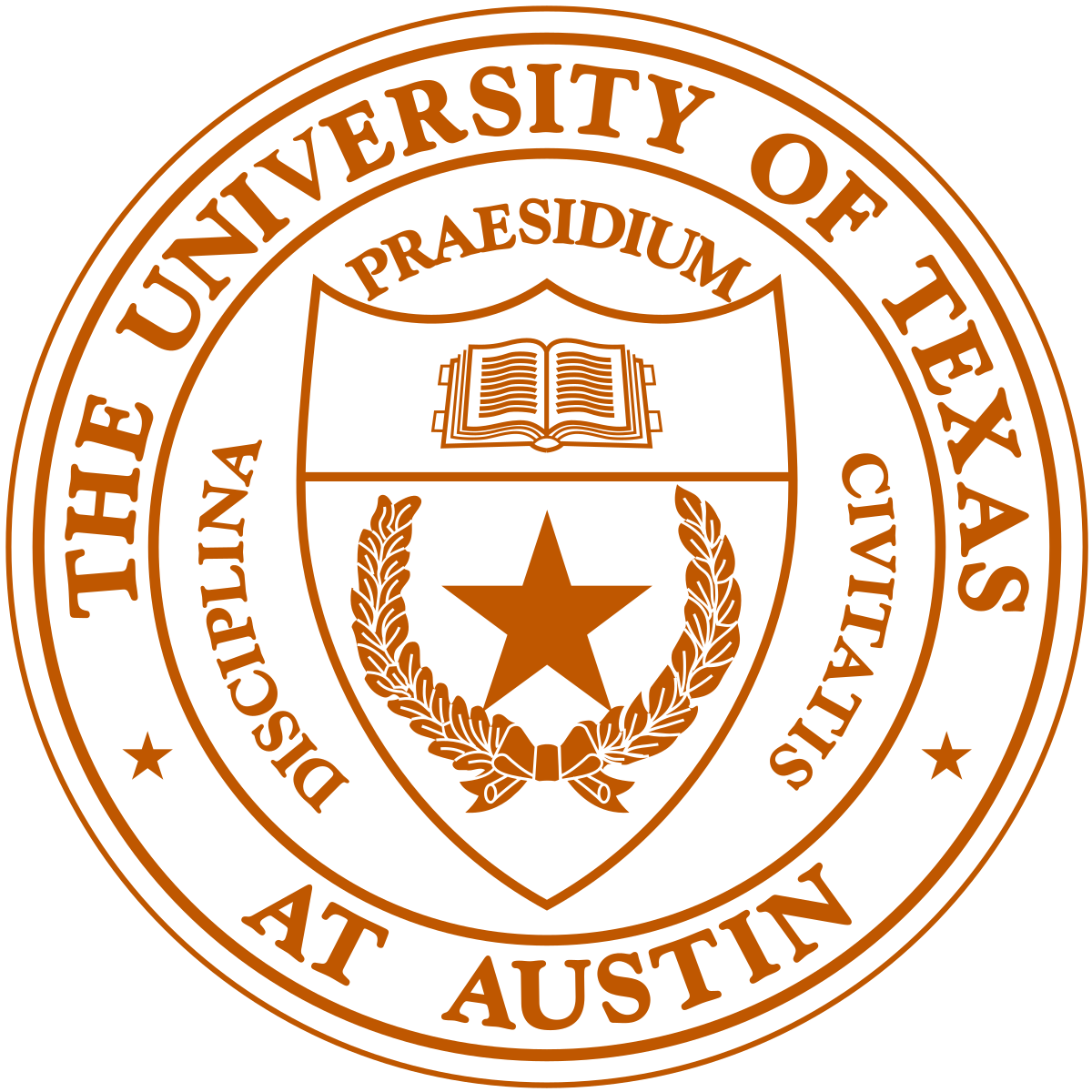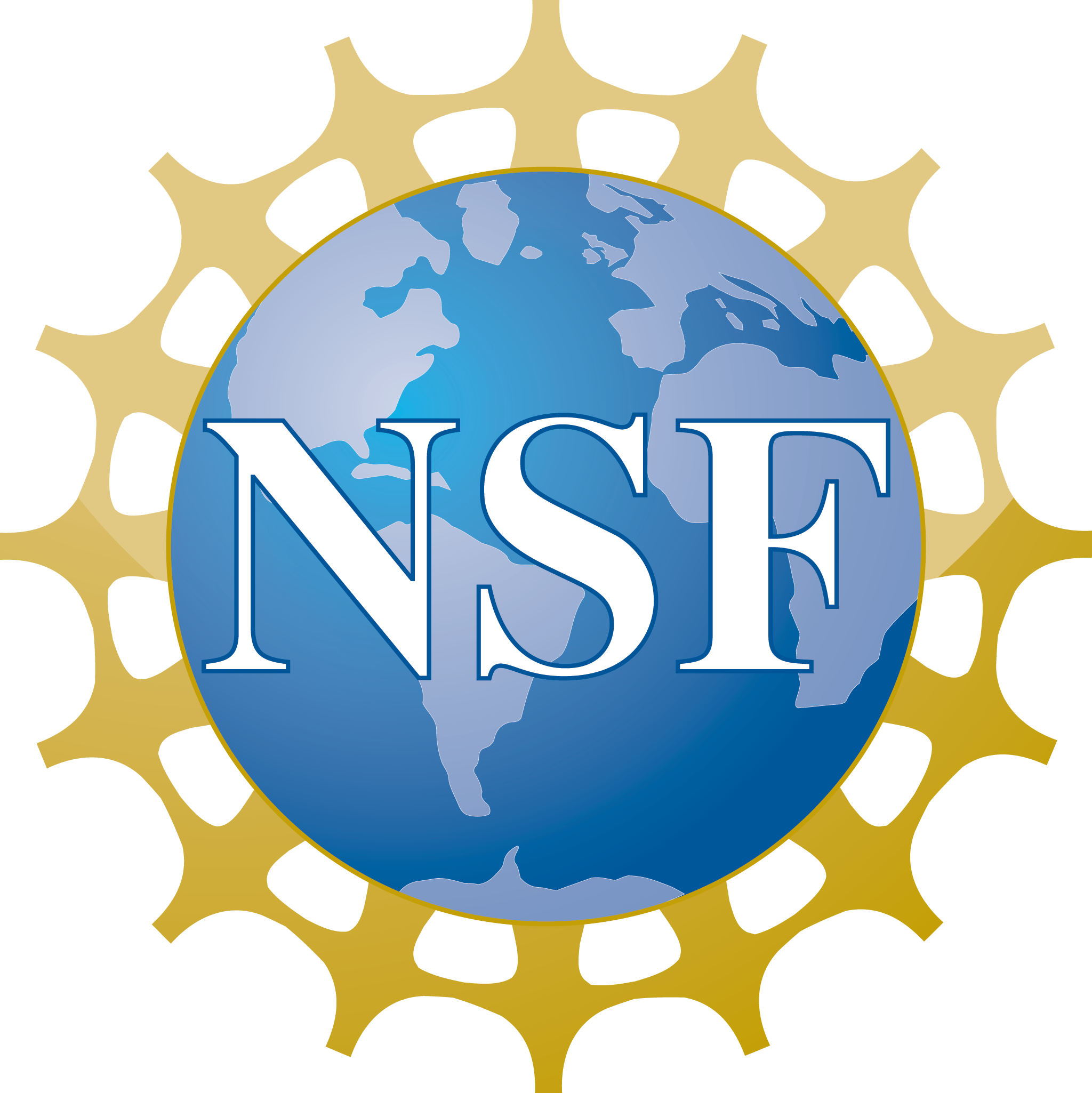Brummer & Partners MathDataLab mini course:

Randomized methods in linear algebra and their applications in data science

Lecturer: Per-Gunnar Martinsson (Univ. of Texas at Austin)

Course objectives: The lectures will describe a set of recently developed randomized algorithms for accelerating matrix computations and the analysis of large data sets. A recurring theme will be the use of randomized embeddings that reduce the effective dimensionality of data sets while in certain respects preserving their geometric properties. We will describe how to use the methods in practice, and how their performance can be analyzed mathematically.

Format: Three lectures delivered via Zoom, at 15:15 - 16:00 (CET), on November 17, 18, 19.

Target audience: The lectures will be self-contained, and will in principle assume only knowledge of basic material on linear algebra and probability theory.

Course timeline/content: See flyer.

Lecture slides: pdf.

Surveys and articles: Several surveys on randomized linear algebra are available, see Section 1.7 of this paper for a partial list. The following papers follow the presentation in these lectures closely:
• E. Liberty, F. Woolfe, P.G. Martinsson, V. Rokhlin, and M. Tygert, "Randomized algorithms for the low-rank approximation of matrices". Proceedings of the National Academy of Sciences, 2007 104: 20167-20172. Local pdf. This is a short paper that succinctly describes the main ideas behind randomized low rank approximation.

• N. Halko, P.G. Martinsson, J. Tropp, "Finding structure with randomness: Probabilistic algorithms for constructing approximate matrix decompositions." SIAM Review, 53(2), 2011, pp. 217-288. Local pdf. This survey describes randomized low rank approximation in detail, including discussions of how to use subspace iteration to improve accuracy, structured random maps to reduce the asymptotic complexity, and single pass algorithms. It contains the full probabilistic error analysis.

• P.G. Martinsson, "Randomized methods for matrix computations." The Mathematics of Data, IAS/Park City Mathematics Series, 25(4), pp. 187 - 231, 2018. Arxiv.org report #1607.01649. Local pdf. This survey is written to be accessible to users from a broad range of backgrounds. It focuses on practical aspects rather than theoretical analysis.

• P.G. Martinsson, "Randomized Projection Methods in Linear Algebra and Data Analysis." SIAM News, December 2018. This is a short and easily accessible news piece written to introduce these methods to a wide audience.

• P.G. Martinsson and J. Tropp, "Randomized Numerical Linear Algebra: Foundations & Algorithms" Acta Numerica, 2020. Arxiv report 2002.01387. Local pdf. This is a long survey that aims at summarizing major findings in the field in the past decade.
Software:
• Tutorial codes implementing RSVD in Matlab. There is GPU support via Matlab (for supported machines).
• Matlab script illustrating a Gaussian embedding. This is the code used in Lecture 1.
• Matlab script generating the error curves in the slides.
• RSVDPACK (With Sergey Voronin.) CPU and GPU implementations in C of most of the techniques covered: RSVD, randomized ID and CUR, etc.
• ID (With Mark Tygert.) FORTRAN and Matlab codes for RSVD, RSFT, interpolative decompositions, etc.
• HQRRP (With Gregorio Quintana-Orti, Nathan Heavner, and Robert van de Geijn.) Highly optimized implementations of (Householder) column pivoted QR with randomization for pivoting. Functions with LAPACK compatible interfaces are included.

Research support by:P.G. Martinsson, November 2020